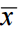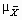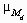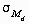### Three distributions

#### Population Distribution:

The population is the whole set of values, or individuals, you are interested in. For example, if you want to know the average height of the residents of India, that is your population, ie, the population of India.

Population characteristic are mean (μ), Standard deviation (σ) , proportion (P) , median,  percentiles etc. The value of a population characteristic is fixed. This characteristics are called population distribution. They are symbolized by Greek characters as they are population parameters.

#### Sample Distribution:

The sample is a subset of the population, and is the set of values you actually use in your estimation. Let’s think 1000 individual you have selected for your study to know about average height of the residents of India. This sample has some quantity computed from values e.g.  mean (x ), Standard deviation (s) , sample proportion etc. This is called sample distribution. The mean and standard deviation are symbolized by Roman characters as they are sample statistics.

#### Sampling Distribution:

Researchers often use a sample to draw inferences about the population that sample is from. To do that, they make use of a probability distribution that is very important in the world of statistics: the sampling distribution. It is theoretical distribution. The distribution of sample statistics is called sampling distribution.We have a population of x values whose histogram is the probability distribution of x.  Select a sample of size n from this population and calculate a sample statistic e.g.. This procedure can be repeated indefinitely and generates a population of values for the sample statistic and the histogram is the sampling distribution of the sample statistics.

For example, If you draw an indefinite number of sample of 1000 respondents from the population the distribution of the infinite number of sample means would be called the sampling distribution of the mean.

Take another example, suppose that a sample of size sixteen (N=16) is taken from some population. The mean of the sixteen numbers is computed. Next a new sample of sixteen is taken, and the mean is again computed. If this process were repeated an infinite number of times, the distribution of the now infinite number of sample means would be called the sampling distribution of the mean.

Consider below diagram to get more clarification about sampling distribution.The population which consists of a set of scores (5, 6, 7, 8 and 9) which distribute around a parameter mean of 7.00. From this population, we can draw a number of samples. Each sample consists of three scores which constitute a subset of the population. The sample scores distribute around some statistic mean for each sample. For sample A, for instance, the scores are 5, 6 and 7 (the sample distribution for A) and the associated statistic mean is 6.00. For sample B the scores are 5, 8 and 8, and the statistic mean is 7.00. Each sample has a statistic mean. The statistics associated with the various samples can now be gathered into a distribution of their own. The distribution will consist of a set of values of a statistic, rather than a set of observed values. This leads to the definition for a sampling distribution: A sampling distribution is a statement of the frequency with which values of statistics are observed or are expected to be observed when a number of random samples is drawn from a given population.

Every statistic has a sampling distribution. For example, suppose that instead of the mean, medians were computed for each sample. The infinite number of medians would be called the sampling distribution of the median.

The sampling distribution of the mean is represented by the symbol, that of the median by, etc.

The standard deviation of the sampling distribution of the mean is called the standard error of the mean and is symbolized by. Similarly, the standard deviation of the sampling distribution of the median is called the standard error of the median and is symbolized by.